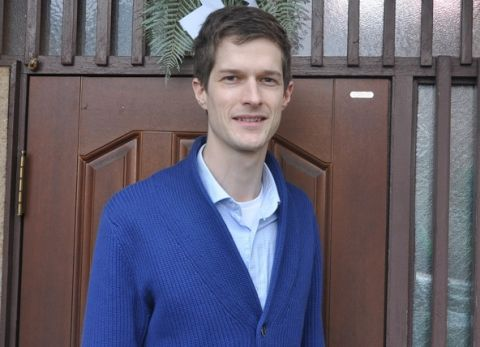# Program for Mathematics

Grant to recruit an international researcher
for a postdoctoral position

Martin Herschend, the Algebra Group

Uppsala University

## Higher Dimensional Representation Theory

Dr Martin Herschend will receive funding from the Knut and Alice Wallenberg Foundation to recruit an international researcher for a postdoctoral position at the Algebra Group at the Department of Mathematics, Uppsala University.

The project will consist of research in representation theory, a branch of pure mathematics which studies mathematical concepts by means of linear algebra. Linear algebra was originated as a method of solving simultaneous systems of linear equations by means of matrices by the great German mathematician Gottfried Wilhelm von Leibniz at the end of 17th century.

Representation theory translates problems into the framework of linear algebra and thereby makes them more accessible. There exist many applications of representation theory within mathematics including algebraic geometry, number theory, and differential equations, as well as in other fields of science and engineering including quantum mechanics and signal processing.

One of abstract mathematical structures studied in representation theory is an object with two operations called algebra. An example of algebra is the set of real numbers with addition and multiplication. The most common tool to study representations of algebras is Auslander-Reiten theory developed in the 1970’s by an American mathematician Maurice Auslander and a Norwegian one, Idun Reiten.

The goal of the project is extending applications of Auslander-Reiten theory to several dimensions. A modified version of the theory introduced a few years ago by the Japanese mathematician Osamu Iyama may be helpful in obtaining such generalizations.